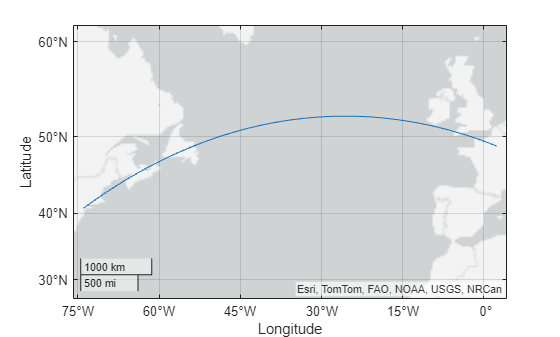# track2

Geographic track points from starting and ending points

## Syntax

``[lattrk,lontrk] = track2(lat1,lon1,lat2,lon2)``
``[lattrk,lontrk] = track2(lat1,lon1,lat2,lon2,units)``
``[lattrk,lontrk] = track2(lat1,lon1,lat2,lon2,ellipsoid)``
``[lattrk,lontrk] = track2(lat1,lon1,lat2,lon2,ellipsoid,units)``
``[lattrk,lontrk] = track2(lat1,lon1,lat2,lon2,ellipsoid,units,npts)``
``[lattrk,lontrk] = track2(method,___)``
``mat = track2(___)``

## Description

### Track Points Along Unit Sphere

example

````[lattrk,lontrk] = track2(lat1,lon1,lat2,lon2)` finds the latitude and longitude coordinates of points along a great circle track from the starting point with coordinates `lat1` and `lon1` to the ending point with coordinates `lat2` and `lon2`. This syntax references the coordinates to a unit sphere and assumes that all input arguments are in degrees.```
````[lattrk,lontrk] = track2(lat1,lon1,lat2,lon2,units)` specifies the angle units `units` for the inputs and outputs.```

### Track Points Along Ellipsoid

example

````[lattrk,lontrk] = track2(lat1,lon1,lat2,lon2,ellipsoid)` finds track points along a geodesic on the reference ellipsoid `ellipsoid`.```
````[lattrk,lontrk] = track2(lat1,lon1,lat2,lon2,ellipsoid,units)` specifies both the reference ellipsoid and the units for the coordinates.```
````[lattrk,lontrk] = track2(lat1,lon1,lat2,lon2,ellipsoid,units,npts)` specifies the number of track points to find.```

example

````[lattrk,lontrk] = track2(method,___)`, where `method` is `"rh"`, calculates track points along rhumb lines. The default for `method` is `"gc"`, which calculates track points along great circles (for spheres) or geodesics (for ellipsoids).```
````mat = track2(___)` returns the latitude and longitude coordinates of the track points in the matrix `mat`.```

## Examples

collapse all

Find points along a great circle track from New York City to Paris.

`[latgc,longc] = track2(40.71,-74.01,48.86,2.35);`

Find points along a rhumb line track between the same cities.

`[latrh,lonrh] = track2("rh",40.71,-74.01,48.86,2.35);`

Compare the great circle and rhumb line tracks by displaying the track points on a map. Use a blue line for the great circle track and a red line for the rhumb line track.

```geoplot(latgc,longc,"b") hold on geoplot(latrh,lonrh,"r")```Create a World Geodetic System of 1984 (WGS84) reference ellipsoid with a length unit of nautical miles.

`wgs84 = wgs84Ellipsoid("nm");`

Find points along a geodesic track from New York City to Paris.

`[lattrk,lontrk] = track2(40.71,-74.01,48.86,2.35,wgs84);`

Display the track on a map.

`geoplot(lattrk,lontrk)`## Input Arguments

collapse all

Latitude of the starting point, specified as a scalar or column vector.

The sizes of `lat1` and `lon1` must match.

To find multiple tracks from a single starting point, specify `lat1` and `lon1` as scalars and `lat2` and `lon2` as column vectors.

Data Types: `single` | `double`

Longitude of the starting point, specified as a scalar or column vector.

The sizes of `lat1` and `lon1` must match.

To find multiple tracks from a single starting point, specify `lat1` and `lon1` as scalars and `lat2` and `lon2` as column vectors.

Data Types: `single` | `double`

Latitude of the ending point, specified as a scalar or column vector.

The sizes of `lat2` and `lon2` must match.

To find multiple tracks from a single starting point, specify `lat1` and `lon1` as scalars and `lat2` and `lon2` as column vectors.

Data Types: `single` | `double`

Longitude of the ending point, specified as a scalar or column vector.

The sizes of `lat2` and `lon2` must match.

To find multiple tracks from a single starting point, specify `lat1` and `lon1` as scalars and `lat2` and `lon2` as column vectors.

Data Types: `single` | `double`

Angle unit, specified as one of these options:

• `"degrees"` — Degrees

• `"radians"` — Radians

Data Types: `char` | `string`

Reference ellipsoid, specified as a `referenceSphere` object, a `referenceEllipsoid` object, an `oblateSpheroid` object, or a two-element vector of the form `[semimajor_axis eccentricity]`, where `semimajor_axis` is the length of the semimajor axis and `eccentricity` is the eccentricity. The values `semimajor_axis` and `eccentricity` must be of data type `double`.

The default value of `[1 0]` represents the unit sphere.

Track method, specified as one of these options:

• `"gc"` — Find track points along a great circle path (for spheres) or a geodesic path (for ellipsoids).

• `"rh"` — Find track points along a rhumb line path.

Data Types: `char` | `string`

Number of track points to include in `lattrk` and `lontrk`, specified as a positive integer.

Data Types: `double`

## Output Arguments

collapse all

Latitude coordinates of the track points, returned as a column vector with `npts` elements or a matrix of size `npts`-by-`length(lat2)`. The function returns `lattrk` as a column vector if `lat2` and `lon2` are scalars, and as a matrix if `lat2` and `lon2` are column vectors.

Longitude coordinates of the track points, returned as a column vector with `npts` elements or a matrix of size `npts`-by-`length(lon2)`. The function returns `lattrk` as a column vector if `lat2` and `lon2` are scalars, and as a matrix if `lat2` and `lon2` are column vectors.

Latitude and longitude coordinates of the track points, returned as a matrix equivalent to `[lattrk lontrk]`.

## Version History

Introduced before R2006a